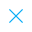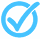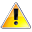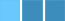Call Support +91-85588-96644

Keep me logged in
You can't leave Captcha Code empty
By submitting this form, you agree to the Terms & Privacy Policy.
ORTests given

OR# Pair of Linear Equations in Two Variables - 7

Questions

Time

Highest
score

Level

## ENGLISH

Language

Topics Covered:Simultaneous equations - linear and quadraticPair of Linear Equations in Two VariablesPair of Linear Equations in Two VariablesPair of Linear Equations in Two VariablesPair of Linear Equations in Two VariablesPair of Linear Equations in Two VariablesPair of Linear Equations in Two VariablesPair of Linear Equations in Two VariablesPair of Linear Equations in Two VariablesPair of Linear Equations in Two VariablesThis is a concept-building practice test and may not have exact structure as you would expect in the actual exam. Please exercise your discretion to attempt it or go to structured Featured Section.

Description:
Pair of Linear Equations in two Variables - 7